# Rotating frame transformation

This tutorial needs review

## Introduction

Rotating frame transformation is often used in NMR spectroscopy to simplify description of magnetization vector in the magnetic field.

Perhaps it is easier to first calculate derivative of a vector rotating in a fixed laboratory frame and after that switch to the case of static vector and rotating frame.

In the end we can allow the vector change in the laboratory frame and get the final formula for derivative in the rotating frame.

## Rotating vector, fixed frame (1)

In fixed frame, imagine vector A rotating with a positive angular frequency ω. Direction of such rotation of A will be by convention - counter-clockwise.

Let's determine derivative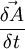$\frac{\vec{\delta A}}{\delta t}$.

First calculate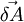$\vec{\delta A}$ - how much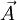$\vec{A}$ changes within time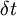$\displaystyle \delta t$: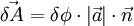$\vec{\delta A} = \delta\phi \cdot |\vec{a}| \cdot \vec{n}$

In the equation above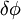$\displaystyle \delta \phi$ - amount of rotation that occurs during time$\displaystyle \delta t$,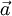$\vec{a}$ - projection of$\vec{A}$ on the plane normal to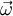$\displaystyle \vec{\omega}$, and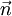$\vec{n}$ - unit vector collinear with$\vec{\delta A}$$\vec{\delta A}$ is essentially the length of the infinitesimal arc drawn by the tip of the vector$\vec{A}$ during the interval$\displaystyle \delta \phi$

## Rotating vector, fixed frame (2)

Notice that$\vec{\delta A}$ is normal to the plane formed by$\vec{\omega}$ and$\vec{A}$.

Rotation angle can be expressed as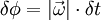$\delta \phi = |\vec{\omega}| \cdot \delta t$

So, we have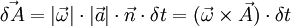$\vec{\delta A} = |\vec{\omega}| \cdot |\vec{a}| \cdot \vec{n} \cdot \delta t = (\vec{\omega}\times\vec{A}) \cdot \delta t$

In the previous step the definition of vector cross product was used. Therefore: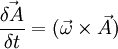$\frac{\vec{\delta A}}{\delta t} = (\vec{\omega}\times\vec{A})$

## Fixed vector, rotating frame

If instead$\vec{A}$ is fixed in the laboratory frame, but the reference frame is rotating in the same direction and rate as above, our derivative of vector within the rotating frame will simply equal above with the minus sign: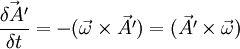$\frac{\vec{\delta A'}}{\delta t} = -(\vec{\omega}\times\vec{A'}) = (\vec{A'}\times\vec{\omega})$

where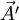$\vec{A'}$ is coordinate of the vector in the rotating frame

## Full rotating frame transformation

Now we have$\vec{A}$ also changing in the static laboratory frame with a derivative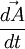$\frac{\vec{d A}}{d t}$.

Then full transformation will look like: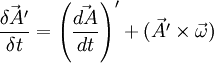$\frac{\vec{\delta A'}}{\delta t} = \left(\frac{\vec{d A}}{d t}\right)' + (\vec{A'}\times\vec{\omega})$

where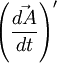$\left(\frac{\vec{d A}}{d t}\right)'$ is$\frac{\vec{d A}}{d t}$, recalculated in the new rotating coordinates.